Courses
Courses for Kids
Free study material
Free LIVE classes
More

# How to Subtract 5-Digit Numbers?## Overview of Subtraction

Last updated date: 15th Mar 2023
Total views: 32.4k
Views today: 0.12k

Subtraction is an arithmetic operation in Mathematics that is used to calculate the difference between different operands, such as whole numbers, integers, fractions, algebraic expressions, and so on. Subtraction is done by taking away or removing things.

When you have a group of objects and you take away a few objects from it, the group becomes smaller and this is what subtraction does. It is a way to reduce numbers, operands, or objects. In this article, we are going to learn 5-digit subtraction with borrowing and we will also know how we can subtract 5-digit numbers.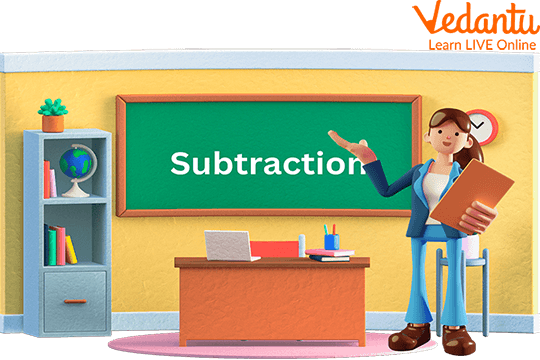Subtraction

## What is Subtraction?

It's a Maths operation in which you take the difference between two numbers. When we subtract the number of things that are in the group, it reduces or becomes less.

### Important Terms for Subtraction

1. Subtrahend

2. Minuend

3. Difference

Subtrahend: Subtrahend means the number which is to be subtracted.

Minuend: It means the number from which another number is to be subtracted.

Difference: It will be the answer or the number which is left after the subtraction.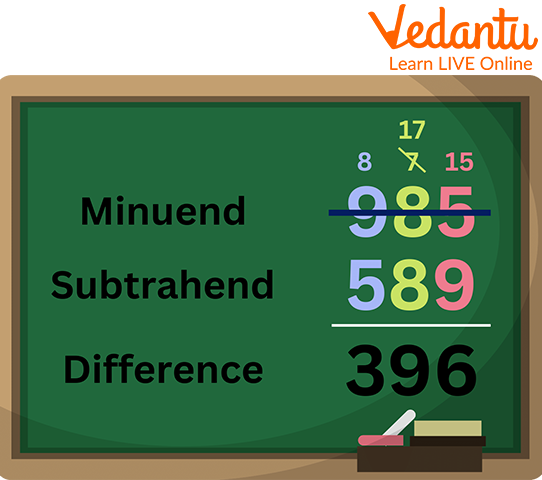Minuend, Subtrahend and Difference

## 5 - Digit Subtraction with Borrowing

It's very easy to learn about subtraction but subtracting a 5-digit number is a little bit difficult. But here we will learn about it in very simple steps.

Step 1. Firstly, you need to arrange the given number in a column. (Ones under ones, tens under tens, hundreds under hundreds, and so on). Here, 51278 - 28051.

Step 2. Then beginning with the ones you go on subtracting columns, borrowing from the next column to the left. Then your answer is there.

At the ones, tens, and hundreds places, we subtract them as

8 - 1 = 7

7 - 5 = 2

2 - 0 =

Here, at the thousand places, we took a borrow and it became 11. So 11 - 8 gives 3. Now, due to borrowing 5 became 4. So 4 - 2 = 2.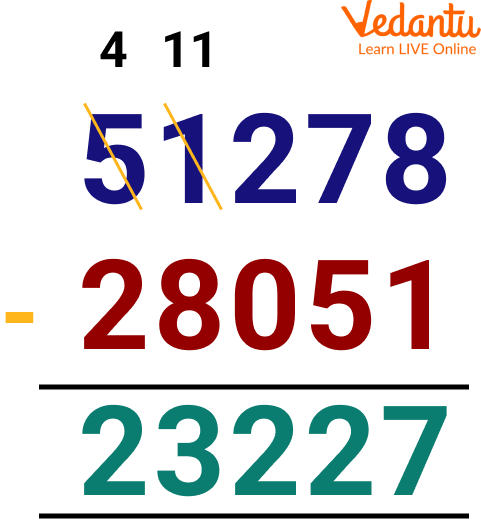Difference Between 51278 and 28051

## Solved Examples

Let’s see some 5-digit subtraction with answers:

Q1. Find the difference between 65633 and 24111

Ans: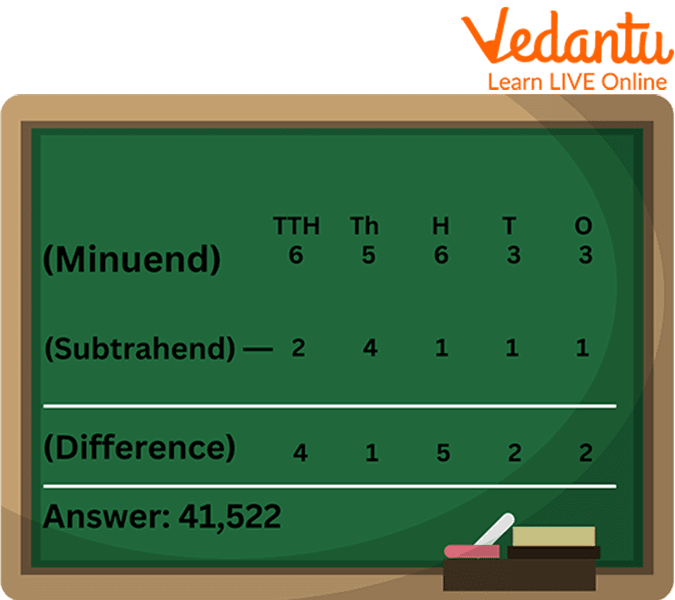Solved Example

Step 1. Arrange the number in a correct manner i.e (ones under ones, tens under tens, hundreds under hundreds, and so on).

Step 2. Subtract the ones $3-1=2$

Step 3. Subtract the tens $3-1=2$

Step 4. Subtract the hundreds $6-1=5$

Step 5. Subtract the thousands $5-4=1$

Step 6. Subtract the ten thousand $6-2=4$

Q2. Find the difference between 79356 and 42143.

Ans.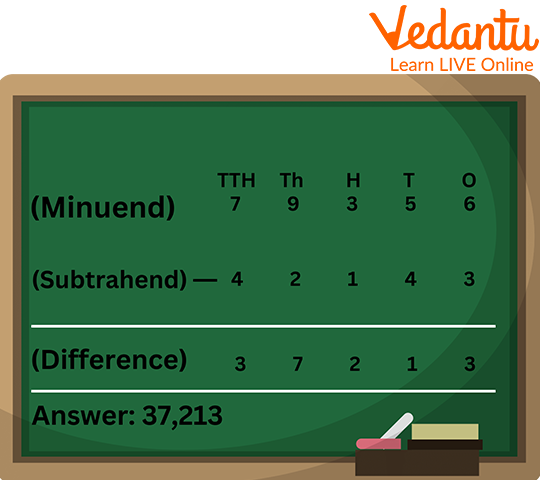Solved Example

Step 1. Arrange the number in a correct manner i.e. (ones under ones, tens under tens, hundreds under hundreds, and so on).

Step 2. Subtract the ones $6-3=3$

Step 3. Subtract the tens $5-4=1$

Step 4. Subtract the hundreds $3-1=2$

Step 5. Subtract the thousands $9-2=7$

Step 6. Subtract the ten thousand $7-4=3$

Q3. Find the difference between 71229 and 34164.

Ans: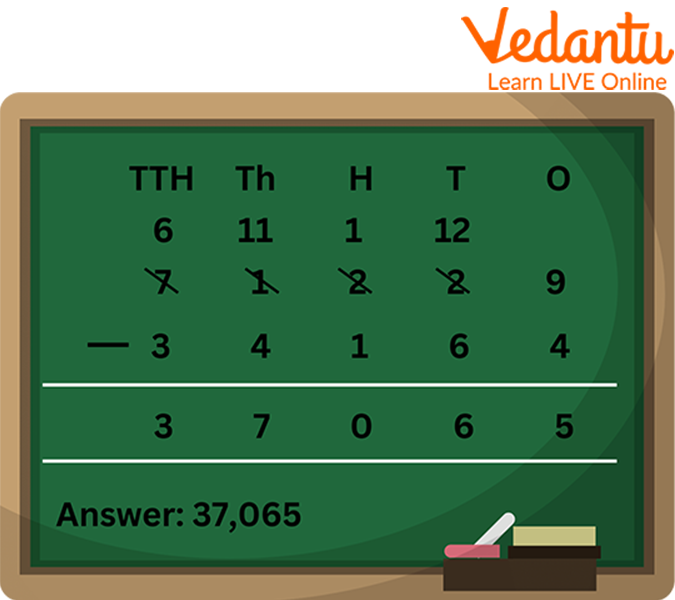Solved Example

Step 1. Subtract the ones $9-4=5$

Step 2. Subtract the tens but here 6 is greater than 2, so we will borrow 1 from hundred, now we have 12 tens. So $12-6=6$

Step 3. Subtract the hundreds $1-1=0$

Step 4. Subtract the thousands but here 4 is greater than 1, so we will borrow 1 from ten thousand. Now, we have 11 thousand. So $11-4=7$

Step 5. Subtract the ten thousand $6-3=3$

## Practice Questions

Subtract the given 5-digit number:

1. $51373-34151$

2. $71543-31215$

3. $34617-13411$

Ans:

1. 17222

2. 40328

3. 21206

## Summary

So, in this article, we learned about subtraction and why it's important to learn subtraction. Simple methods to subtract 5-digit numbers and effective tips were also shared. Every operation of Maths is not only used in our everyday life but also develops our minds. It's a type of mental exercise. We hope to have helped you grasp the concept of subtracting 5-digit numbers. Visit our website to download worksheets of the same.

## FAQs on How to Subtract 5-Digit Numbers?

Q1. What are the types of subtraction?

Ans: The types of subtraction mean the various methods used in subtraction. For example, subtraction with and without regrouping, subtraction using number charts, subtraction using number lines, the subtraction of small numbers using your fingers, and so on.

Q2. Where do we use subtraction?

Ans: Subtraction is used in our day-to-day life. For example, if we want to know how much money we spent on the items that we bought, or how much money is left with us, or if we want to calculate the time left in finishing a task, we use subtraction.

Q3. Is subtraction commutative?

Ans. The commutative property states that the numbers on which we operate can be moved or swapped from their position without making any difference to the answer. The property holds for addition and multiplication, but not for subtraction and division.